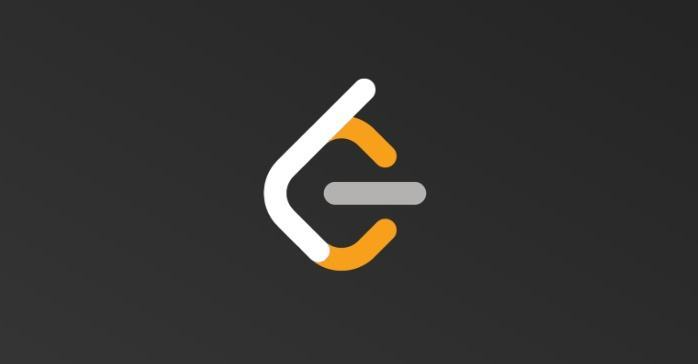# LeetCode680- 验证回文字符串 Ⅱ-Easy示例1：输入: "aba"输出: True示例2：输入: "abca"输出: True解释: 你可以删除c字符

• 遍历字符串中的每一个字符时，获取第一个字符和最后一个字符，下标分别为：$i 和$j

• 如果这两个位置的字符相等，则 $i=$i+1,$j=$j-1，再次比较字符是否相等，直到循环终止条件 $i>$j

• 如果这两个位置的字符不相等，则依次去掉低位的字符和高位的字符。去掉低位字符，则此时起始位为 $k=$i+1,高位为 $t=$j

• 去掉高位字符，则此时起始位为 $k=$i,高位为 $t=$j-1

• 如果去掉低位和高位后，有一个能够成为回文字符串，则整个字符串为回文字符串

• 时间复杂度：O(n)

<?phpclass Solution {    /**    * @param Integer[] $nums * @return Integer */ public function maxProduct($nums) {      $len = strlen($s);      for ($i=0,$j=$len-1;$i < $j;$i++, $j--) { if ($s[$i] ==$s[$j]) continue;$flag1 = $flag2 = true; for ($k = $i+1,$t=$j;$k < $t;$k++, $t--) { if ($s[$k] !=$s[$t]) {$flag1 = false;        break;      }    }      for ($k =$i, $t=$j-1; $k <$t; $k++,$t--) {        if ($s[$k] != $s[$t]) {          $flag2 = false; break; } } return$flag1 || \$flag2;    }    return true;}## 评论